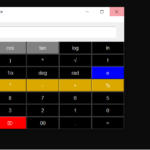Scientific GUI Calculator In Python With Source Code

The Scientific GUI Calculator In Python is a simple project developed using Python. The project file contains a python script (scientific-cal.py). This is a simple GUI-based project which is very easy to understand and use. Also, this project makes a conv...

Type : Project

File Size : 11.9 KBSimilar Projects and Reports

Scientific GUI Calculator In Python With Source Code

The Scientific GUI Calculator In Python is a simple project developed using Python. The project file contains a python script (scientific-cal.py). This is a simple GUI-based project which is very easy to understand and use. Also, this project makes a conv...

Scientific Calculator In Python With Source Code

The Scientific Calculator In Python is a simple project developed using Python. The project file contains a python script (my_Calculator.py). This is a simple GUI-base project which is very easy to understand and use. Also, this project makes a convenient...

GUI CALCULATOR IN PYTHON WITH SOURCE CODE

The GUI Calculator In Python is a simple project developed using Python. The project contains the numbers, operators, and sign like in normal calculator. So, the user cannot enter the number themselves, they can just click on the numbers they want in the ...

Calculator With GUI In Python With Source Code

The Calculator With GUI In Python is a simple project developed using Python. The project contains the numbers, operators, and signs like in a normal calculator. So, the user cannot enter the number themselves, they can just click on the numbers they want...

Python Calculator In GUI With Source Code

The Python Calculator In GUI is a simple project developed using Python. The project contains the numbers, operators, and signs like on a normal calculator. Here, the user can just click on the numbers they want in the calculations. Hence, the user can us...

CALCULATOR IN PYTHON WITH SOURCE CODE

This Calculator project In Python is a simple project developed using Python. The project contains the numbers, operators, and sign like in normal calculator. So, the user can enter any number they want in the calculations. Hence, the user can use a simpl...